# User:Tohline/SSC/Structure/PowerLawDensity

(diff) ← Older revision | Current revision (diff) | Newer revision → (diff)

# Power-Law Density Distributions

Here we begin with the same second-order, one-dimensional ODE that governs the structure of polytropic spheres, namely, the

Lane-Emden Equation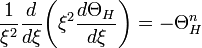$\frac{1}{\xi^2} \frac{d}{d\xi}\biggl( \xi^2 \frac{d\Theta_H}{d\xi} \biggr) = - \Theta_H^n$ ,

and examine whether or not this governing relation can be satisfied by a power-law enthalpy distribution of the form,

ΘH = Aξ − α,

where A and α are assumed to be constants. We note, up front, that such a solution will not satisfy the boundary conditions that are imposed on polytropic spheres. But the simplistic form of a power-law solution can nevertheless sometimes be instructive.

## Derivation

Plugging the power-law expression for the dimensionless enthalpy into both sides of the Lane-Emden equation gives,

− α(1 − α)Aξ − (2 + α) = − Anξ − αn.

Hence, the power-law enthalpy distribution works as long as,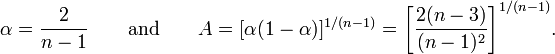$\alpha = \frac{2}{n-1} ~~~~~~\mathrm{and}~~~~~~ A = [\alpha (1 -\alpha)]^{1/(n-1)} = \biggl[ \frac{2(n-3)}{(n-1)^2} \biggr]^{1/(n-1)}.$

This means that hydrostatic balance can be established at all radial positions within a spherically symmetric configuration for power-law density distributions of the form,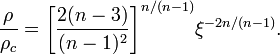$\frac{\rho}{\rho_c} = \biggl[ \frac{2(n-3)}{(n-1)^2} \biggr]^{n/(n-1)} \xi^{- 2n/(n-1)}.$

(Note that, in this case, the subscript c should not represent the central conditions but, rather, conditions at some characteristic radial position within the configuration.) As has been mentioned in our accompanying discussion of analytically defined structures of isolated polytropes, this expression for the power-law density profile can be found in the introductory section of the article by Patryk Mach (2012, J. Math. Phys., 53, 062503).

## Examples

It looks like the derived solution makes some physical sense only for polytropic indices n > 3. For n = 4, the relevant power-law density distribution is,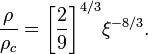$\frac{\rho}{\rho_c} = \biggl[ \frac{2}{9} \biggr]^{4/3} \xi^{- 8/3}.$

For n = (3 + ε) and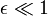$\epsilon \ll 1$,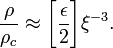$\frac{\rho}{\rho_c} \approx \biggl[ \frac{\epsilon}{2} \biggr] \xi^{- 3}.$

For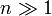$n \gg 1$,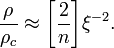$\frac{\rho}{\rho_c} \approx \biggl[ \frac{2}{n} \biggr] \xi^{- 2}.$

Hence, for polytropic indices in the range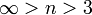$\infty > n > 3$, the relevant power-law density distribution lies between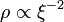$\rho \propto \xi^{-2}$ and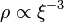$\rho \propto \xi^{-3}$.

## Isothermal Equation of State

Suppose the gas is isothermal so that the relevant equation of state is,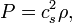$P = c_s^2 \rho ,$

where cs is the sound speed. To determine what power-law density distribution will satisfy hydrostatic equilibrium in this case, it is better to return to the original statement of hydrostatic balance for spherically symmetric configurations,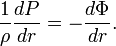$\frac{1}{\rho} \frac{dP}{dr} = -\frac{d\Phi}{dr} .$

Plugging in the isothermal equation of state and assuming a radial density distribution of the form,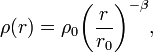$\rho(r) = \rho_0 \biggl( \frac{r}{r_0} \biggr)^{-\beta} ,$

we obtain,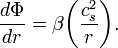$\frac{d\Phi}{dr} = \beta \biggl(\frac{c_s^2}{r}\biggr) .$

Therefore, the Poisson equation gives,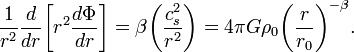$\frac{1}{r^2}\frac{d}{dr}\biggl[r^2 \frac{d\Phi}{dr}\biggr] = \beta \biggl(\frac{c_s^2}{r^2}\biggr)= 4\pi G \rho_0 \biggl( \frac{r}{r_0} \biggr)^{-\beta} .$

This relation can be satisfied only if,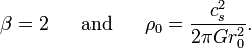$\beta = 2 ~~~~~\mathrm{and}~~~~~ \rho_0 = \frac{c_s^2}{2\pi G r_0^2} .$

Hence, hydrostatic balance can be achieved for an isothermal gas with a power-law density distribution of the form,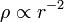$\rho \propto r^{-2}$. Because an isothermal P(ρ) equation of state is obtained by setting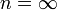$n = \infty$ in the more general polytropic equation of state, the result just derived is consistent with the above, more general analysis which showed that, for values of the polytropic index$n \gg 1$, the equilibrium power-law density distribution tends toward a$\rho \propto r^{-2}$ distribution.

# Related Wikipedia Discussions

 © 2014 - 2020 by Joel E. Tohline |   H_Book Home   |   YouTube   | Appendices: | Equations | Variables | References | Ramblings | Images | myphys.lsu | ADS |